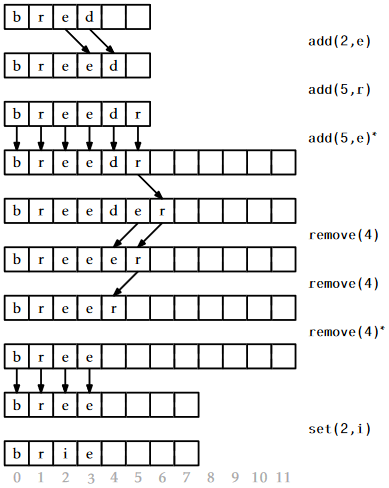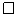# 2.1: ArrayStack - Fast Stack Operations Using an Array

$$\newcommand{\vecs}{\overset { \rightharpoonup} {\mathbf{#1}} }$$ $$\newcommand{\vecd}{\overset{-\!-\!\rightharpoonup}{\vphantom{a}\smash {#1}}}$$$$\newcommand{\id}{\mathrm{id}}$$ $$\newcommand{\Span}{\mathrm{span}}$$ $$\newcommand{\kernel}{\mathrm{null}\,}$$ $$\newcommand{\range}{\mathrm{range}\,}$$ $$\newcommand{\RealPart}{\mathrm{Re}}$$ $$\newcommand{\ImaginaryPart}{\mathrm{Im}}$$ $$\newcommand{\Argument}{\mathrm{Arg}}$$ $$\newcommand{\norm}{\| #1 \|}$$ $$\newcommand{\inner}{\langle #1, #2 \rangle}$$ $$\newcommand{\Span}{\mathrm{span}}$$ $$\newcommand{\id}{\mathrm{id}}$$ $$\newcommand{\Span}{\mathrm{span}}$$ $$\newcommand{\kernel}{\mathrm{null}\,}$$ $$\newcommand{\range}{\mathrm{range}\,}$$ $$\newcommand{\RealPart}{\mathrm{Re}}$$ $$\newcommand{\ImaginaryPart}{\mathrm{Im}}$$ $$\newcommand{\Argument}{\mathrm{Arg}}$$ $$\newcommand{\norm}{\| #1 \|}$$ $$\newcommand{\inner}{\langle #1, #2 \rangle}$$ $$\newcommand{\Span}{\mathrm{span}}$$$$\newcommand{\AA}{\unicode[.8,0]{x212B}}$$

An ArrayStack implements the list interface using an array $$\mathtt{a}$$, called the backing array. The list element with index $$\mathtt{i}$$ is stored in $$\mathtt{a[i]}$$. At most times, $$\mathtt{a}$$ is larger than strictly necessary, so an integer $$\mathtt{n}$$ is used to keep track of the number of elements actually stored in $$\mathtt{a}$$. In this way, the list elements are stored in $$\mathtt{a}$$,..., $$\mathtt{a[n-1]}$$ and, at all times, $$\texttt{a.length} \ge \mathtt{n}$$.

    T[] a;
int n;
int size() {
return n;
}


## $$\PageIndex{1}$$ The Basics

Accessing and modifying the elements of an ArrayStack using $$\mathtt{get(i)}$$ and $$\mathtt{set(i,x)}$$ is trivial. After performing any necessary bounds-checking we simply return or set, respectively, $$\mathtt{a[i]}$$.

    T get(int i) {
if (i < 0 || i > n - 1) throw new IndexOutOfBoundsException();
return a[i];
}
T set(int i, T x) {
if (i < 0 || i > n - 1) throw new IndexOutOfBoundsException();
T y = a[i];
a[i] = x;
return y;
}


The operations of adding and removing elements from an ArrayStack are illustrated in Figure $$\PageIndex{1}$$. To implement the $$\mathtt{add(i,x)}$$ operation, we first check if $$\mathtt{a}$$ is already full. If so, we call the method $$\mathtt{resize()}$$ to increase the size of $$\mathtt{a}$$. How $$\mathtt{resize()}$$ is implemented will be discussed later. For now, it is sufficient to know that, after a call to $$\mathtt{resize()}$$, we can be sure that $$\texttt{a.length}> \mathtt{n}$$. With this out of the way, we now shift the elements $$\mathtt{a[i]},\ldots,\mathtt{a[n-1]}$$ right by one position to make room for $$\mathtt{x}$$, set $$\mathtt{a[i]}$$ equal to $$\mathtt{x}$$, and increment $$\mathtt{n}$$.Figure $$\PageIndex{1}$$: A sequence of $$\mathtt{add(i,x)}$$ and $$\mathtt{remove(i)}$$ operations on an ArrayStack. Arrows denote elements being copied. Operations that result in a call to $$\mathtt{resize()}$$ are marked with an asterisk.
    void add(int i, T x) {
if (i < 0 || i > n) throw new IndexOutOfBoundsException();
if (n + 1 > a.length) resize();
for (int j = n; j > i; j--)
a[j] = a[j-1];
a[i] = x;
n++;
}


If we ignore the cost of the potential call to $$\mathtt{resize()}$$, then the cost of the $$\mathtt{add(i,x)}$$ operation is proportional to the number of elements we have to shift to make room for $$\mathtt{x}$$. Therefore the cost of this operation (ignoring the cost of resizing $$\mathtt{a}$$) is $$O(\mathtt{n}-\mathtt{i})$$.

Implementing the $$\mathtt{remove(i)}$$ operation is similar. We shift the elements $$\mathtt{a[i+1]},\ldots,\mathtt{a[n-1]}$$ left by one position (overwriting $$\mathtt{a[i]}$$) and decrease the value of $$\mathtt{n}$$. After doing this, we check if $$\mathtt{n}$$ is getting much smaller than $$\texttt{a.length}$$ by checking if $$\texttt{a.length} \ge 3\mathtt{n}$$. If so, then we call $$\mathtt{resize()}$$ to reduce the size of $$\mathtt{a}$$.

    T remove(int i) {
if (i < 0 || i > n - 1) throw new IndexOutOfBoundsException();
T x = a[i];
for (int j = i; j < n-1; j++)
a[j] = a[j+1];
n--;
if (a.length >= 3*n) resize();
return x;
}


If we ignore the cost of the $$\mathtt{resize()}$$ method, the cost of a $$\mathtt{remove(i)}$$ operation is proportional to the number of elements we shift, which is $$O(\mathtt{n}-\mathtt{i})$$.

## $$\PageIndex{2}$$ Growing and Shrinking

The $$\mathtt{resize()}$$ method is fairly straightforward; it allocates a new array $$\mathtt{b}$$ whose size is $$2\mathtt{n}$$ and copies the $$\mathtt{n}$$ elements of $$\mathtt{a}$$ into the first $$\mathtt{n}$$ positions in $$\mathtt{b}$$, and then sets $$\mathtt{a}$$ to $$\mathtt{b}$$. Thus, after a call to $$\mathtt{resize()}$$, $$\texttt{a.length} = 2\mathtt{n}$$.

    void resize() {
T[] b = newArray(Math.max(n*2,1));
for (int i = 0; i < n; i++) {
b[i] = a[i];
}
a = b;
}


Analyzing the actual cost of the $$\mathtt{resize()}$$ operation is easy. It allocates an array $$\mathtt{b}$$ of size $$2\mathtt{n}$$ and copies the $$\mathtt{n}$$ elements of $$\mathtt{a}$$ into $$\mathtt{b}$$. This takes $$O(\mathtt{n})$$ time.

The running time analysis from the previous section ignored the cost of calls to $$\mathtt{resize()}$$. In this section we analyze this cost using a technique known as amortized analysis. This technique does not try to determine the cost of resizing during each individual $$\mathtt{add(i,x)}$$ and $$\mathtt{remove(i)}$$ operation. Instead, it considers the cost of all calls to $$\mathtt{resize()}$$ during a sequence of $$m$$ calls to $$\mathtt{add(i,x)}$$ or $$\mathtt{remove(i)}$$. In particular, we will show:

Lemma $$\PageIndex{1}$$.

If an empty ArrayStack is created and any sequence of $$m\ge 1$$ calls to $$\mathtt{add(i,x)}$$ and $$\mathtt{remove(i)}$$ are performed, then the total time spent during all calls to $$\mathtt{resize()}$$ is $$O(m)$$.

Proof. We will show that any time $$\mathtt{resize()}$$ is called, the number of calls to $$\mathtt{add}$$ or $$\mathtt{remove}$$ since the last call to $$\mathtt{resize()}$$ is at least $$\mathtt{n}/2-1$$. Therefore, if $$\mathtt{n}_i$$ denotes the value of $$\mathtt{n}$$ during the $$i$$th call to $$\mathtt{resize()}$$ and $$r$$ denotes the number of calls to $$\mathtt{resize()}$$, then the total number of calls to $$\mathtt{add(i,x)}$$ or $$\mathtt{remove(i)}$$ is at least

$\sum_{i=1}^{r} (\mathtt{n}_i/2-1) \le m \enspace , \nonumber$

which is equivalent to

$\sum_{i=1}^{r} \mathtt{n}_i \le 2m + 2r \enspace . \nonumber$

On the other hand, the total time spent during all calls to $$\mathtt{resize()}$$ is

$\sum_{i=1}^{r} O(\mathtt{n}_i) \le O(m+r) = O(m) \enspace , \nonumber$

since $$r$$ is not more than $$m$$. All that remains is to show that the number of calls to $$\mathtt{add(i,x)}$$ or $$\mathtt{remove(i)}$$ between the $$(i-1)$$th and the $$i$$th call to $$\mathtt{resize()}$$ is at least $$\mathtt{n}_i/2$$.

There are two cases to consider. In the first case, $$\mathtt{resize()}$$ is being called by $$\mathtt{add(i,x)}$$ because the backing array $$\mathtt{a}$$ is full, i.e., $$\texttt{a.length} = \mathtt{n}=\mathtt{n}_i$$. Consider the previous call to $$\mathtt{resize()}$$: after this previous call, the size of $$\mathtt{a}$$ was $$\texttt{a.length}$$, but the number of elements stored in $$\mathtt{a}$$ was at most $$\texttt{a.length}/2=\mathtt{n}_i/2$$. But now the number of elements stored in $$\mathtt{a}$$ is $$\mathtt{n}_i=\texttt{a.length}$$, so there must have been at least $$\mathtt{n}_i/2$$ calls to $$\mathtt{add(i,x)}$$ since the previous call to $$\mathtt{resize()}$$.

The second case occurs when $$\mathtt{resize()}$$ is being called by $$\mathtt{remove(i)}$$ because $$\texttt{a.length} \ge 3\mathtt{n}=3\mathtt{n}_i$$. Again, after the previous call to $$\mathtt{resize()}$$ the number of elements stored in $$\mathtt{a}$$ was at least $$\mathtt{a.length/2}-1$$.1 Now there are $$\mathtt{n}_i\le\texttt{a.length}/3$$ elements stored in $$\mathtt{a}$$. Therefore, the number of $$\mathtt{remove(i)}$$ operations since the last call to $$\mathtt{resize()}$$ is at least

\begin{align} R &\ge \texttt{a.length}/2 - 1 - \texttt{a.length}/3\nonumber\\ &= \texttt{a.length}/6 - 1\nonumber\\ &= (\texttt{a.length}/3)/2 - 1\nonumber\\ &\ge \mathtt{n}_i/2 -1\enspace .\nonumber \end{align}\nonumber

In either case, the number of calls to $$\mathtt{add(i,x)}$$ or $$\mathtt{remove(i)}$$ that occur between the $$(i-1)$$th call to $$\mathtt{resize()}$$ and the $$i$$th call to $$\mathtt{resize()}$$ is at least $$\mathtt{n}_i/2-1$$, as required to complete the proof.## $$\PageIndex{3}$$ Summary

The following theorem summarizes the performance of an ArrayStack:

Theorem $$\PageIndex{1}$$.

An ArrayStack implements the List interface. Ignoring the cost of calls to $$\mathtt{resize()}$$, an ArrayStack supports the operations

• $$\mathtt{get(i)}$$ and $$\mathtt{set(i,x)}$$ in $$O(1)$$ time per operation; and
• $$\mathtt{add(i,x)}$$ and $$\mathtt{remove(i)}$$ in $$O(1+\mathtt{n}-\mathtt{i})$$ time per operation.

Furthermore, beginning with an empty ArrayStack and performing any sequence of $$m$$ $$\mathtt{add(i,x)}$$ and $$\mathtt{remove(i)}$$ operations results in a total of $$O(m)$$ time spent during all calls to $$\mathtt{resize()}$$.

The ArrayStack is an efficient way to implement a Stack. In particular, we can implement $$\mathtt{push(x)}$$ as $$\mathtt{add(n,x)}$$ and $$\mathtt{pop()}$$ as $$\mathtt{remove(n-1)}$$, in which case these operations will run in $$O(1)$$ amortized time.

#### Footnotes

1The $${}-1$$ in this formula accounts for the special case that occurs when $$\mathtt{n}=0$$ and $$\texttt{a.length} = 1$$.

This page titled 2.1: ArrayStack - Fast Stack Operations Using an Array is shared under a CC BY license and was authored, remixed, and/or curated by Pat Morin (Athabasca University Press) .